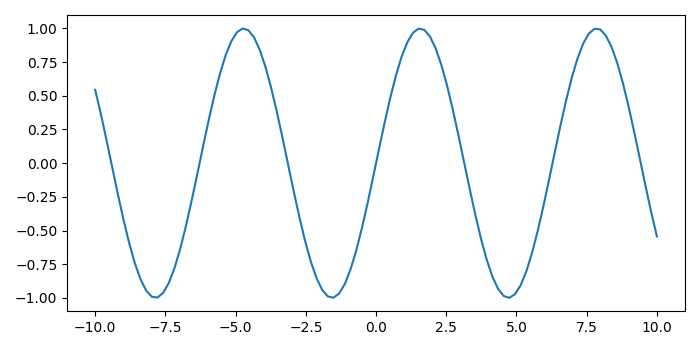# Matplotlib â€“ How to show the coordinates of a point upon mouse click?

To create a custom mouse cursor in matplotlib, we can take the following steps

## Steps

• Set the figure size and adjust the padding between and around the subplots.

• Create a new figure or activate an existing figure.

• Bind the function *mouse_event* to the event *button_press_event*.

• Create x and y data points using numpy.

• Plot the x and y data points using plot() method.

• To display the figure, use Show() method.

## Example

import numpy as np
from matplotlib import pyplot as plt

plt.rcParams["figure.figsize"] = [7.00, 3.50]
plt.rcParams["figure.autolayout"] = True

def mouse_event(event):
print('x: {} and y: {}'.format(event.xdata, event.ydata))

fig = plt.figure()
cid = fig.canvas.mpl_connect('button_press_event', mouse_event)

x = np.linspace(-10, 10, 100)
y = np.sin(x)

plt.plot(x, y)

plt.show()


## Output

It will produce the following output −Now, click different points on the plot and it will show their coordinates on the console.

x: -3.099305446290094 and y: -0.013811108549791173
x: -0.2865652183685867 and y: -0.2067543563498595
x: -3.0280968329249927 and y: -0.1844916739113902
x: -5.7696284474814 and y: 0.4240216460734405
x: -3.9182044999887626 and y: 0.6837529411889172